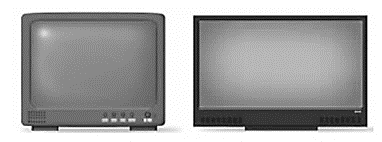# the heights of the both TV screen.### Precalculus: Mathematics for Calcu...

6th Edition
Stewart + 5 others
Publisher: Cengage Learning
ISBN: 9780840068071### Precalculus: Mathematics for Calcu...

6th Edition
Stewart + 5 others
Publisher: Cengage Learning
ISBN: 9780840068071

#### Solutions

Chapter 1.6, Problem 86E
To determine

## To find: the heights of the both TV screen.

Expert Solution

h+5=32.41inch and 1.8h=49.33inch .

### Explanation of Solution

Given:

Two television monitors sitting side by side on a shelf in an appliances store have the same screen height.Calculation:

Width of the convectional screen is h+5 .

Width of the wide screen is 1.8h .

Therefore, diagonal length of convection screen is h2+(h+5)2.....(1) .

And the diagonal length of wide screen is h2+(1.8h)2.....(2) .

According to the question, diagonal length of wide screen is 14 inches more than convection screen.

h2+(1.8h)2=14+h2+(h+5)2h2+(1.8h)2=196+h2+(h+5)2+28h2+(h+5)2after simplifying,h=27.41 inches

Therefore, height of conventional screen and wides screen are:

h+5=32.41inch and 1.8h=49.33inch respectively.

### Have a homework question?

Subscribe to bartleby learn! Ask subject matter experts 30 homework questions each month. Plus, you’ll have access to millions of step-by-step textbook answers!